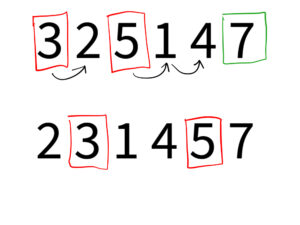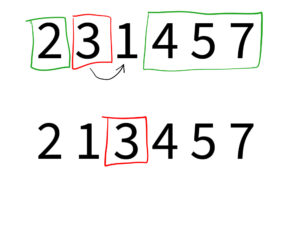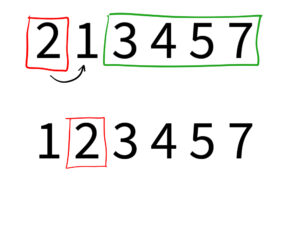# Bubble sort algorithm in C#### Bubble sort algorithm in C#

Bubble sort is one of the simplest sorting algorithms in computer science and is often used as an introduction to the concept of sorting. Despite its simplicity, bubble sort can be quite effective for small-sized data sets, and it is a great algorithm for teaching and learning the basics of sorting. The algorithm repeatedly steps through the list to be sorted, compares each pair of adjacent items and swaps them if they are in the wrong order. The pass through the list is repeated until no swaps are needed, which indicates that the list is sorted.

In C#, it is possible to implement the bubble sort algorithm using the built-in Array and List classes. The following is an example of how to implement the bubble sort algorithm using an array of integers:

FLASH SALES
\$29.95
NEW
\$249.00
Become a C# PRO and land your first developer job!

In this example, the outer loop iterates through the array, while the inner loop iterates through the sub-array of the remaining unsorted elements.

If the current element is greater than the next element, the elements are swapped. This process is repeated until the entire array is sorted.

### Visualization

The image below shows you 4 iterations of the main loop that have to be done to sort the array from our example above.

• green rectangles are untouched parts of the array (if such a part appears during the iteration);
• red rectangles it the value to be moved;
• arrows show us the movement of the value;
• on the next line, you will see the array after this iteration;

This image shows us the very first iteration of our outer loop. You can see that 3 will stay in the same place as it is smaller than 5. However, 5 and 7 will be moved to the right for one and two places, respectively.

``

The second iteration will move 3 and 5 for one and two positions, respectively. But you should remember that each move is nothing else but a swap where the bigger number goes to the right and the smaller one to the left.

``

At this stage, you can already see that half of our array has been sorted {…4, 5, 7}, and only 3 will be swapped with 1.

``

And the last iteration performers just the one final swap.

``````

### Summary

In conclusion, bubble sort is a simple and easy-to-understand sorting algorithm that can be effectively implemented in C# using the built-in Array and List classes. It is a great choice for small data sets, and it is an excellent algorithm for teaching and learning the basics of sorting.

Get to know more about built-in sorting algorithms in C#.